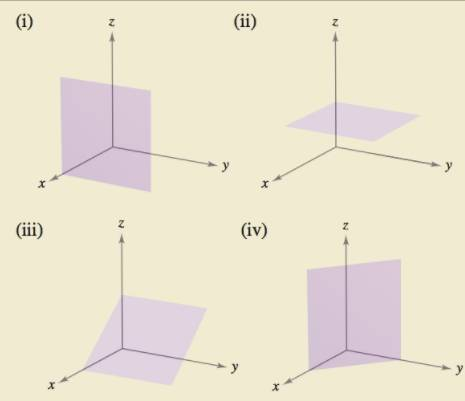Chapter 11.5, Problem 100E

Chapter
Section
Textbook Problem

# HOW DO YOU SEE IT? Match the general equation with its graph. Then state what axis or plane the equation is parallel to.(a) a x + b y + d = 0 (b) a x + d = 0 (c) c z + d = 0 (d) a x + c z + d = 0(a)

To determine
For given equation of plane ax+by+d=0 find the graph that matches the equation.

Explanation

Given:

The provided equation of plane is:

ax+by+d=0

The following graph is:

Explanation:

As we know the provided equation contains x and y variables but it does not contain z variable

(b)

To determine
For given equation of plane ax+by+d=0 find the graph that matches the equation of plane..

(c)

To determine
For given equation of plane ax+by+d=0 find the graph that matches the equation of plane

(d)

To determine
For given equation of plane ax+by+d=0 find the graph that matches the equation of plane.

### Still sussing out bartleby?

Check out a sample textbook solution.

See a sample solution

#### The Solution to Your Study Problems

Bartleby provides explanations to thousands of textbook problems written by our experts, many with advanced degrees!

Get Started